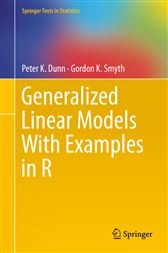Generalized Linear Models With Examples in R

Series: Springer Texts in StatisticsUS\$89.31
ISBNs
• 9781441901170
• 9781441901187

This textbook presents an introduction to generalized linear models, complete with real-world data sets and practice problems, making it applicable for both beginning and advanced students of applied statistics. Generalized linear models (GLMs) are powerful tools in applied statistics that extend the ideas of multiple linear regression and analysis of variance to include response variables that are not normally distributed. As such, GLMs can model a wide variety of data types including counts, proportions, and binary outcomes or positive quantities.

The book is designed with the student in mind, making it suitable for self-study or a structured course. Beginning with an introduction to linear regression, the book also devotes time to advanced topics not typically included in introductory textbooks. It features chapter introductions and summaries, clear examples, and many practice problems, all carefully designed to balance theory and practice. The text also provides a working knowledge of applied statistical practice through the extensive use of R, which is integrated into the text.

Other features include:

•             Advanced topics such as power variance functions, saddlepoint approximations, likelihood score tests, modified profile likelihood, small-dispersion asymptotics, and randomized quantile residuals

•             Nearly 100 data sets in the companion R package GLMsData

•             Examples that are cross-referenced to the companion data set, allowing readers to load the data and follow the analysis in their own R session

• Springer New York; November 2018
• ISBN: 9781441901187
• Read online, or download in DRM-free PDF (digitally watermarked) format
• Title: Generalized Linear Models With Examples in R
• Series: Springer Texts in Statistics
• Author: Peter K. Dunn; Gordon K. Smyth
• Imprint: Springer
ISBNs
• 9781441901170
• 9781441901187